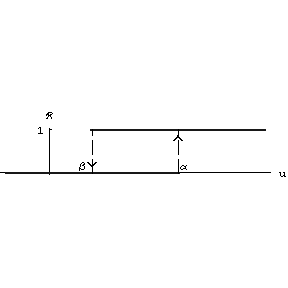# Non-ideal relay

The hysteresis non-linearity denoted by ${\mathcal R} ( \alpha, \beta )$, with thresholds $\alpha$ and $\beta$, and defined for a continuous input $u ( t )$, $t \geq t _ {0}$, and an initial state $r _ {0} \in \{ 0,1 \}$ by the formulas (see Fig.a1.)

$${\mathcal R} ( r _ {0} ; \alpha, \beta ) u ( t ) = \left \{ \begin{array}{ll} r _ {0} &\textrm{ if } \alpha < u ( s ) < \beta, t _ {0} \leq s \leq t, \\ 0 &\textrm{ if either } u ( t ) \leq \beta \textrm{ or } \\ {} & u ( t ) \in ( \beta, \alpha ) \textrm{ and } u ( \tau ) = \beta, \\ 1 &\textrm{ if either } u ( t ) \geq \alpha \textrm{ or } \\ {} & u ( t ) \in ( \beta, \alpha ) \textrm{ and } u ( \tau ) = \alpha, \\ \end{array} \right .$$

where $\tau = \sup \{ s : {s \leq t, u ( s ) = \beta \textrm{ or } u ( s ) = \alpha } \}$, that is, $\tau$ denotes the last switching moment. The input–output operators ${\mathcal R} ( r _ {0} ; \alpha, \beta )$ are discontinuous in the usual function spaces. These operators are monotone in a natural sense, which allows one to use the powerful methods of the theory of semi-ordered spaces in the analysis of systems with non-ideal relays.Figure: n110060a

Non-ideal relay

The Preisach–Giltay model of ferromagnetic hysteresis is described as the spectral decomposition in a continual system of non-ideal relays in the following way. Let $\mu ( \alpha, \beta )$ be a finite Borel measure in the half-plane $\Pi = \{ {( \alpha, \beta ) } : {\alpha > \beta } \}$. The input–output operators of the Preisach–Giltay hysteresis non-linearity at a given continuous input $u ( t )$, $t \geq t _ {0}$, and initial state $S ( t _ {0} )$ is defined by the formula

$$x ( t ) = \int\limits { {\mathcal R} ( r _ {0} ( \alpha, \beta ) ; \alpha, \beta ) u ( t ) } {d \mu ( \alpha, \beta ) } ,$$

where the measurable function $r _ {0} ( \alpha, \beta )$ describes the internal state of the non-linearity at the initial moment $t = t _ {0}$. In contrast to the individual non-ideal relay, the operators of a Preisach–Giltay non-linearity are continuous in the space of continuous functions, provided that the measure $\mu ( \alpha, \beta )$ is absolutely continuous with respect to the Lebesgue measure (cf. Absolute continuity). For detailed properties of Preisach–Giltay hysteresis and further generalizations see [a1], [a2] and the references therein.